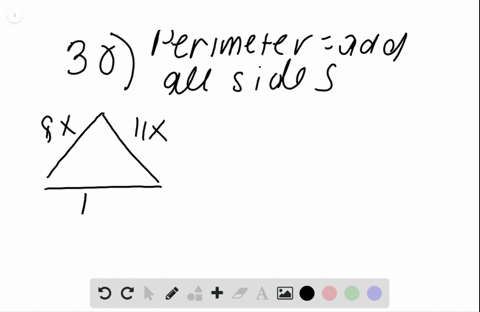Enroll in one of our FREE online STEM bootcamps. Join today and start acing your classes!View Bootcamps### The perimeter of a triangle is 132 $\mathrm{cm}$ …

02:03Problem 29

# In Exercises $24-29$ find the measure of each angle. The measures of the angles of a hexagon are in the ratio $4 : 5 : 5 : 8 : 9 : 9 .$

## Discussion

You must be signed in to discuss.

## Video Transcript

We know that hexagons add up to 720 we're left with 7 20 equals 40 acts. Acts equals 18 multiply 458 and nine by the result angle one of 72.5 angled to is 90 angles three s nine d angle for this 1 44 angle five is 1 62 angle sex is 1 62